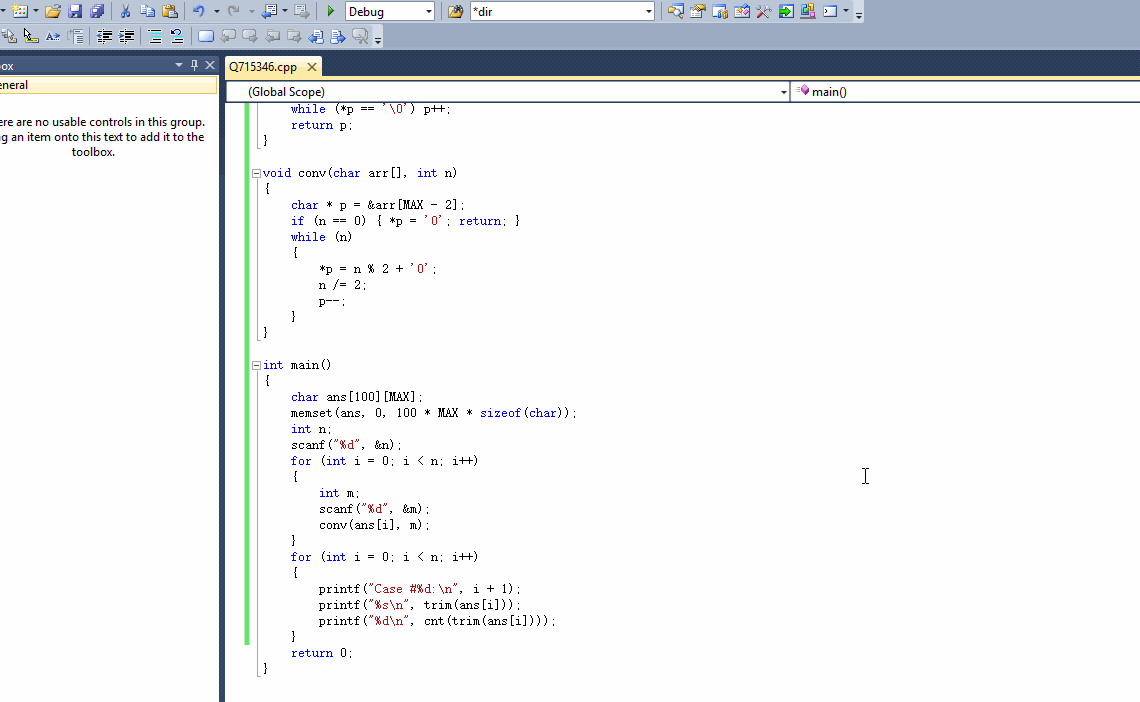###### weixin_43866982

2018-11-30 15:49 阅读 959

# 2018ACM新生赛（热身赛）

3
6
66
666

Case #1:
110
2
Case #2:
1000010
2
Case #3:
1010011010
5

• 点赞
• 写回答
• 关注问题
• 收藏
• 复制链接分享

#### 2条回答默认 最新

• ``````T = int(input())
for i in range(T):
n = int(input())
n_bin = bin(a)[2:]
print(n_bin)
print(len(n_bin) - len(n_bin.replace('1', '')))

``````
点赞 评论 复制链接分享
• # 如果问题得到解决，请点我回答左上角的采纳和向上的箭头，谢谢

``````#include "stdio.h"
#include "string.h"

#define MAX 21

int cnt(char * s)
{
int n = 0;
while (*s != '\0')
{
if (*s == '1')
n++;
s++;
}
return n;
}

char* trim(char * s)
{
char *p = s;
while (*p == '\0') p++;
return p;
}

void conv(char arr[], int n)
{
char * p = &arr[MAX - 2];
if (n == 0) { *p = '0'; return; }
while (n)
{
*p = n % 2 + '0';
n /= 2;
p--;
}
}

int main()
{
char ans[MAX];
memset(ans, 0, 100 * MAX * sizeof(char));
int n;
scanf("%d", &n);
for (int i = 0; i < n; i++)
{
int m;
scanf("%d", &m);
conv(ans[i], m);
}
for (int i = 0; i < n; i++)
{
printf("Case #%d:\n", i + 1);
printf("%s\n", trim(ans[i]));
printf("%d\n", cnt(trim(ans[i])));
}
return 0;
}
``````点赞 评论 复制链接分享